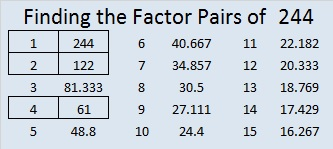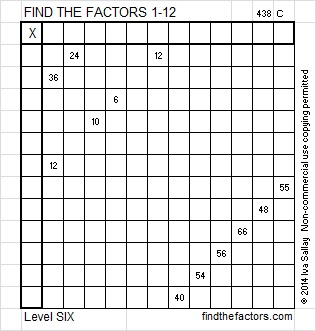# 244 and Level 6

• 244 is a composite number.
• Prime factorization: 244 = 2 x 2 x 61, which can be written 244 = (2^2) x 61
• The exponents in the prime factorization are 2 and 1. Adding one to each and multiplying we get (2 + 1)(1 + 1) = 3 x 2 = 6. Therefore 244 has 6 factors.
• Factors of 244: 1, 2, 4, 61, 122, 244
• Factor pairs: 244 = 1 x 244, 2 x 122, 4 x 61
• Taking the factor pair with the largest square number factor, we get √244 = (√4)(√61) = 2√61 ≈ 15.6205Print the puzzles or type the factors on this excel file: 12 Factors 2014-09-22This site uses Akismet to reduce spam. Learn how your comment data is processed.